Download Presentation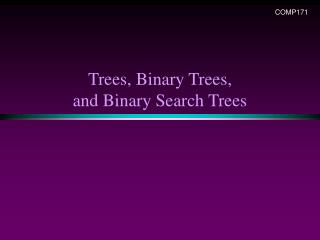Trees, Binary Trees, and Binary Search Trees

# Trees, Binary Trees, and Binary Search Trees - PowerPoint PPT Presentation

COMP171. Trees, Binary Trees, and Binary Search Trees. Trees. Linear access time of linked lists is prohibitive Does there exist any simple data structure for which the running time of most operations (search, insert, delete) is O(log N)? Trees Basic concepts Tree traversal Binary treeI am the owner, or an agent authorized to act on behalf of the owner, of the copyrighted work described.
Download Presentation## Trees, Binary Trees, and Binary Search Trees

An Image/Link below is provided (as is) to download presentation

Download Policy: Content on the Website is provided to you AS IS for your information and personal use and may not be sold / licensed / shared on other websites without getting consent from its author.While downloading, if for some reason you are not able to download a presentation, the publisher may have deleted the file from their server.

- - - - - - - - - - - - - - - - - - - - - - - - - - E N D - - - - - - - - - - - - - - - - - - - - - - - - - -
Presentation Transcript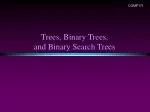COMP171

### Trees, Binary Trees, and Binary Search Trees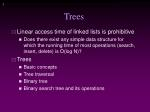Trees
• Linear access time of linked lists is prohibitive
• Does there exist any simple data structure for which the running time of most operations (search, insert, delete) is O(log N)?
• Trees
• Basic concepts
• Tree traversal
• Binary tree
• Binary search tree and its operations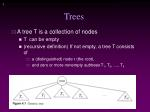Trees
• A tree T is a collection of nodes
• T can be empty
• (recursive definition) If not empty, a tree T consists of
• a (distinguished) node r (the root),
• and zero or more nonempty subtrees T1, T2, ...., Tk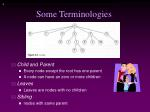Some Terminologies
• Child and Parent
• Every node except the root has one parent
• A node can have an zero or more children
• Leaves
• Leaves are nodes with no children
• Sibling
• nodes with same parent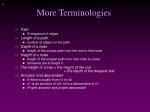More Terminologies
• Path
• A sequence of edges
• Length of a path
• number of edges on the path
• Depth of a node
• length of the unique path from the root to that node
• Height of a node
• length of the longest path from that node to a leaf
• all leaves are at height 0
• The height of a tree = the height of the root = the depth of the deepest leaf
• Ancestor and descendant
• If there is a path from n1 to n2
• n1 is an ancestor of n2, n2 is a descendant of n1
• Proper ancestor and proper descendant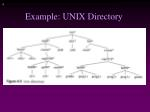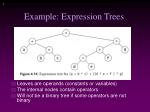Example: Expression Trees
• Leaves are operands (constants or variables)
• The internal nodes contain operators
• Will not be a binary tree if some operators are not binary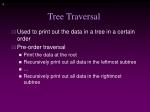Tree Traversal
• Used to print out the data in a tree in a certain order
• Pre-order traversal
• Print the data at the root
• Recursively print out all data in the leftmost subtree
• Recursively print out all data in the rightmost subtree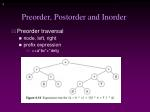Preorder, Postorder and Inorder
• Preorder traversal
• node, left, right
• prefix expression
• ++a*bc*+*defg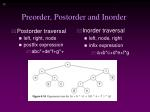Postorder traversal

left, right, node

postfix expression

abc*+de*f+g*+

Inorder traversal

left, node, right

infix expression

a+b*c+d*e+f*g

Preorder, Postorder and InorderBinary Trees
• A tree in which no node can have more than two children
• The depth of an “average” binary tree is considerably smaller than N, even though in the worst case, the depth can be as large as N – 1.

Generic binary tree

Worst-casebinary tree• Possible operations on the Binary Tree ADT
• Parent, left_child, right_child, sibling, root, etc
• Implementation
• Because a binary tree has at most two children, we can keep direct pointers to them
• a linked list is physically a pointer, so is a tree.
• Define a Binary Tree ADT later …A drawing of linked list with one pointer …

A drawing of binary tree with two pointers …

Struct BinaryNode {

double element; // the data

BinaryNode* left; // left child

BinaryNode* right; // right child

}Binary Search Trees (BST)
• A data structure for efficient searching, inser-tion and deletion
• Binary search tree property
• For every node X
• All the keys in its left subtree are smaller than the key value in X
• All the keys in its right subtree are larger than the key value in XBinary Search Trees

A binary search tree

Not a binary search treeBinary Search Trees

The same set of keys may have different BSTs

• Average depth of a node is O(log N)
• Maximum depth of a node is O(N)Searching BST
• If we are searching for 15, then we are done.
• If we are searching for a key < 15, then we should search in the left subtree.
• If we are searching for a key > 15, then we should search in the right subtree.Searching (Find)
• Find X: return a pointer to the node that has key X, or NULL if there is no such node
• Time complexity: O(height of the tree)

find(const double x, BinaryNode* t) constInorder Traversal of BST
• Inorder traversal of BST prints out all the keys in sorted order

Inorder: 2, 3, 4, 6, 7, 9, 13, 15, 17, 18, 20findMin/ findMax
• Goal: return the node containing the smallest (largest) key in the tree
• Algorithm: Start at the root and go left (right) as long as there is a left (right) child. The stopping point is the smallest (largest) element
• Time complexity = O(height of the tree)

BinaryNode* findMin(BinaryNode* t) constInsertion
• Proceed down the tree as you would with a find
• If X is found, do nothing (or update something)
• Otherwise, insert X at the last spot on the path traversed
• Time complexity = O(height of the tree)void insert(double x, BinaryNode*& t)

{

if (t==NULL) t = new BinaryNode(x,NULL,NULL);

else if (x<t->element) insert(x,t->left);

else if (t->element<x) insert(x,t->right);

else ; // do nothing

}Deletion
• When we delete a node, we need to consider how we take care of the children of the deleted node.
• This has to be done such that the property of the search tree is maintained.Deletion under Different Cases
• Case 1: the node is a leaf
• Delete it immediately
• Case 2: the node has one child
• Adjust a pointer from the parent to bypass that nodeDeletion Case 3
• Case 3: the node has 2 children
• Replace the key of that node with the minimum element at the right subtree
• Delete that minimum element
• Has either no child or only right child because if it has a left child, that left child would be smaller and would have been chosen. So invoke case 1 or 2.
• Time complexity = O(height of the tree)void remove(double x, BinaryNode*& t)

{

if (t==NULL) return;

if (x<t->element) remove(x,t->left);

else if (t->element < x) remove (x, t->right);

else if (t->left != NULL && t->right != NULL) // two children

{

t->element = finMin(t->right) ->element;

remove(t->element,t->right);

}

else

{

Binarynode* oldNode = t;

t = (t->left != NULL) ? t->left : t->right;

delete oldNode;

}

}For a generic (binary) tree:

Struct Node {

double element; // the data

Node* left; // left child

Node* right; // right child

}

class Tree {

public:

Tree(); // constructor

Tree(const Tree& t);

~Tree(); // destructor

bool empty() const;

double root(); // decomposition (access functions)

Tree& left();

Tree& right();

void insert(const double x); // compose x into a tree

void remove(const double x); // decompose x from a tree

private:

Node* root;

}

access,

selection

update

(insert and remove are different from those of BST)For BST tree:

Struct Node {

double element; // the data

Node* left; // left child

Node* right; // right child

}

class BST {

public:

BST(); // constructor

BST(const Tree& t);

~BST(); // destructor

bool empty() const;

double root(); // decomposition (access functions)

BST left();

BST right();

bool serch(const double x); // search an element

void insert(const double x); // compose x into a tree

void remove(const double x); // decompose x from a tree

private:

Node* root;

}

access,

selection

update

BST is for efficient search, insertion and removal, so restricting these functions.Weiss textbook:

class BST {

public:

BST();

BST(const Tree& t);

~BST();

bool empty() const;

bool search(const double x); // contains

void insert(const double x); // compose x into a tree

void remove(const double x); // decompose x from a tree

private:

Struct Node {

double element;

Node* left;

Node* right;

Node(…) {…}; // constructuro for Node

}

Node* root;

void insert(const double x, Node*& t) const; // recursive function

void remove(…)

Node* findMin(Node* t);

void makeEmpty(Node*& t); // recursive ‘destructor’

bool contains(const double x, Node* t) const;

}root, left subtree, right subtree are missing:

1. we can’t write other tree algorithms, is implementation dependent,

BUT,

2. this is only for BST (we only need search, insert and remove, may not need other tree algorithms)

so it’s two layers, the public for BST, and the private for Binary Tree.

3. it might be defined internally in ‘private’ part (actually it’s implicitly done).A public non-recursive member function:

void insert(double x)

{

insert(x,root);

}

A private recursive member function:

void insert(double x, BinaryNode*& t)

{

if (t==NULL) t = new BinaryNode(x,NULL,NULL);

else if (x<t->element) insert(x,t->left);

else if (t->element<x) insert(x,t->right);

else ; // do nothing

}